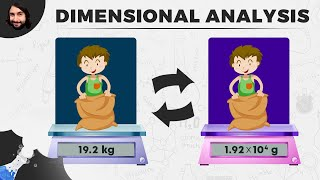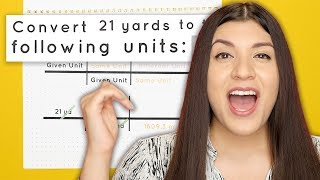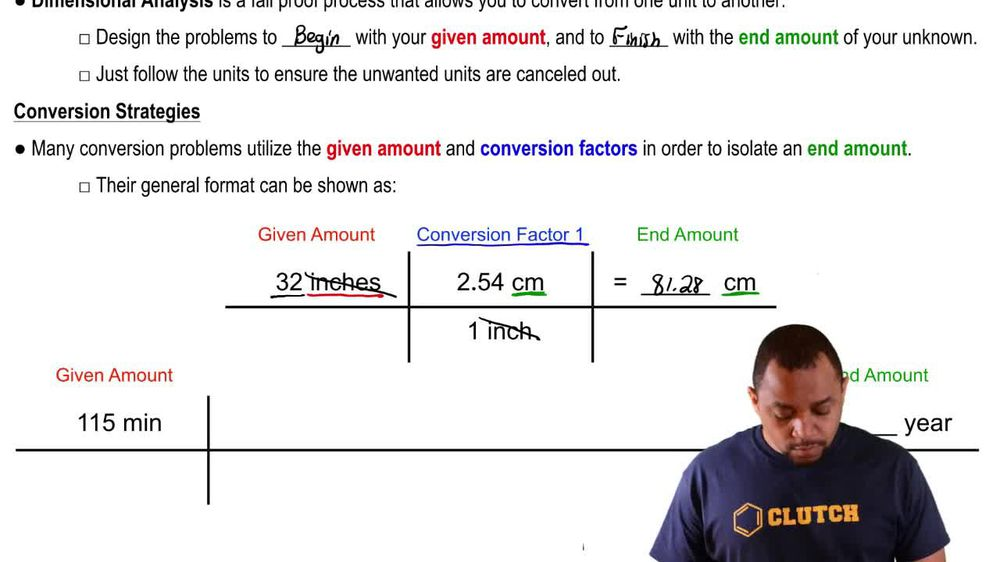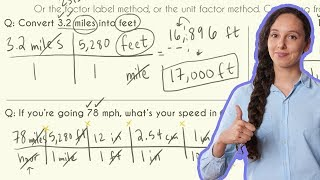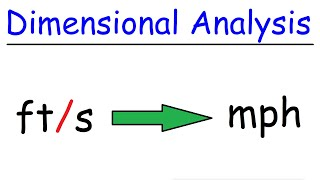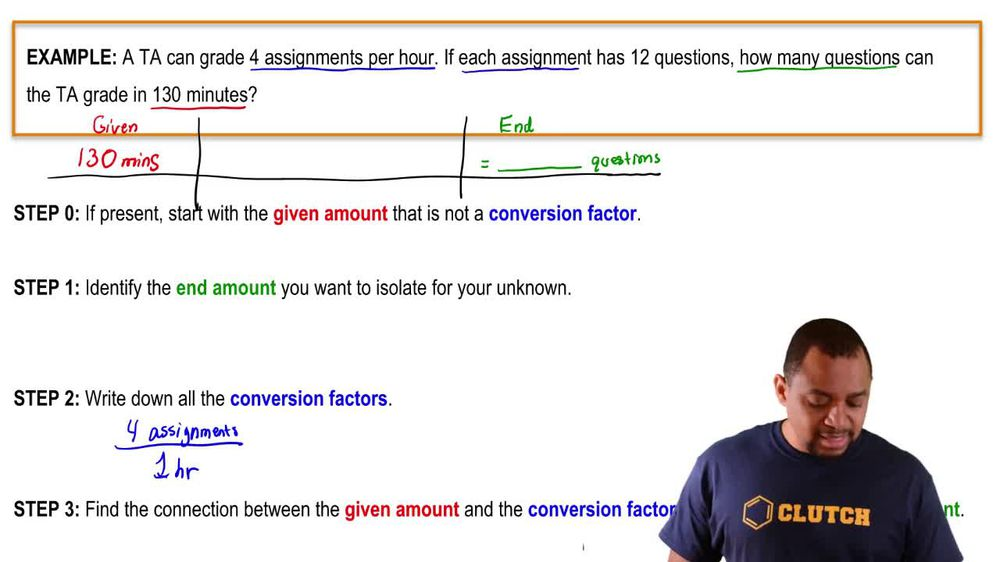Start typing, then use the up and down arrows to select an option from the list.
1. 1. Intro to General Chemistry2. Dimensional Analysis
Problem

# Ocean currents are measured in Sverdrups (sv) where 1 sv = 109 m3/s. The Gulf Stream off the tip of Florida, for instance, has a flow of 35 sv. (b) What mass of water in the Gulf Stream flows past a given point in 24 hours? The density of seawater is 1.025 g/mL.

Relevant Solution2m
Play a video:
Hello everyone today. We have the following problem. A hypothetical measurement termed 50 one's fifth is equal to 14 m cubed per minute is used to measure water currents. If a freshwater river with a density of 1.8 g per milliliter has a flow of nine point 8/5. What mass of freshwater flows past a given point in 18 hours. So some important conversion factors that we have to be aware about is that one hour is equal to 60 minutes. one m cubed is equal to 1000 l And one leader is equal to 1000 militia leaders. And so our overall goal is to convert our fifth to our meters cubed per minute. So we're going to do as we are going to try and find our meters cubed per minute first. So we're gonna take our nine point 8/5. And since we know that one of these fists Is equal to 14 m cubed per minute. When our units cancel out, we're left with 137.2 m cubed per minute. And so that's our first step. Our 2nd step is to take our time And convert that into ultimately g because we want the mass of freshwater. So we have 18 hours are given, We use the conversion factor that 60 minutes equals one hour. We also have the conversion that 137.2 m cubed is equal to one minute. This gets rid of our units of minutes. We can go ahead and multiply by the conversion factor. Next that one m cubed is equal to 1000 liters as stated before this gets rid of our meters cubed. And then lastly, we can multiply by our density was 1.8 g per mila leader. And so we still have to get rid of these leaders and milliliters so we can just multiply by the conversion factor that 1000 mL equals one leader. And so this is going to get rid of our volume. We are going to be left with 1. times 10 to the 11th grams as our final answer. Overall, I hope this helped and until next time.